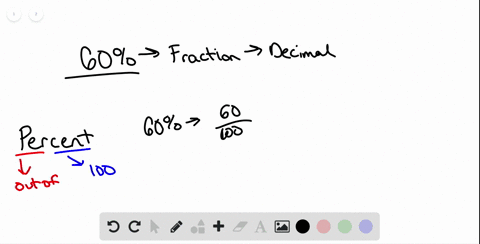Sign up for our free STEM online summer camps starting June 1st!View Summer Courses### In the following exercises, convert each percent …

01:23Need more help? Fill out this quick form to get professional live tutoring.

Get live tutoring
Problem 46

In the following exercises, convert each percent to
(a) a simplified fraction and (b) a decimal

Javier will choose one digit at random from 0 through 9 . The probability he will choose 3 is 10$\%$ .

\begin{aligned} \text { a. As a fraction: } & \\ \qquad 10 \%=& \frac{10}{100} \\ \text { b. As a decimal: } & \\ 10 \% &=10 \\ &=0.10 \end{aligned}

## Discussion

You must be signed in to discuss.

## Video Transcript

So you're 10% and we want to turn that into a fraction and then simplify it on and then also turn it into a decibel. So if we have 10% percent means per 100 so I can turn that into the Fraction 10 over 100 or 10 hundreds Now, in order to simplify and we need to find the greatest common factor, the largest number of those come both be divided by and we since they both ended zero we can see those can both be divided by 10 because it's a fraction. Whatever we do to the numerator, we have to do the denominator. So we end up with a simplify infraction of 1 10 Now, when we have a denominator of 100 we can turn that directly into a decimals. We have 10 hundreds. We can make our decimals say the same thing. We have the tense place and the hundreds place 10 hundreds. Another way to look at this is in 10%. Technically, the decimal point is right here to go from a percent to a decimal. We move the decimal 0.2 places to the left. So again we still end up with 10 hundreds